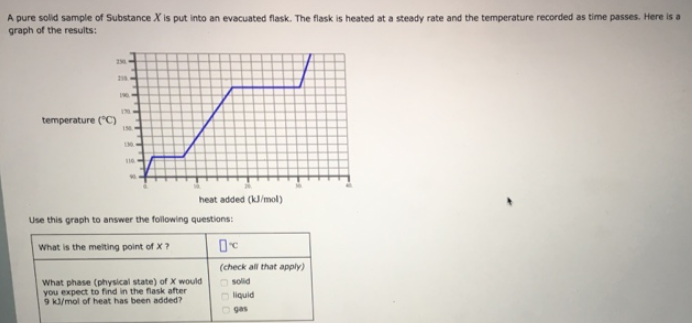# Problem: A pure solid sample of Substance X is put into an evacuated flask. The flask is heated at a steady rate and the temperature recorded as time passes. Here is a graph of the results: Use this graph to answer the following questions:What is the melting point of X?What phase (physical state) of X would you expect to find in the flask after 9 kJ/mol of heat has been added? (check all that apply)            • solid            • liquid             • gas

###### FREE Expert Solution
95% (67 ratings)###### Problem Details

A pure solid sample of Substance X is put into an evacuated flask. The flask is heated at a steady rate and the temperature recorded as time passes. Here is a graph of the results:

Use this graph to answer the following questions:

What is the melting point of X?

What phase (physical state) of X would you expect to find in the flask after 9 kJ/mol of heat has been added? (check all that apply)

• solid

• liquid

• gas